# Math Lesson for Grade 3: Finding Area using a Model of the Distributive Property

Lesson Objective: The lesson is aligned to the Common Core State Standards for Mathematics – 3.MD.7c Geometric Measurement – Use area models to represent the distributive property.

Materials Required: graph paper, calculator

## Lesson Procedure: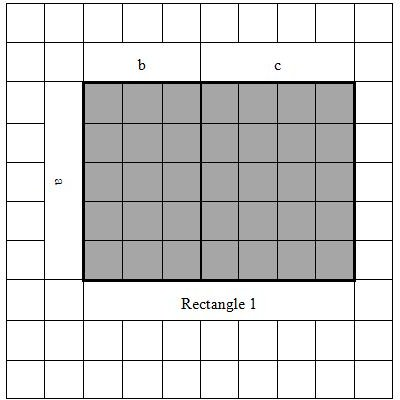Finding Area of Rectangular Figures using Distributive Property

Look at Rectangle 1 shown on the grid. Rectangle 1 has Side a and Side (b + c). Side length of each square on all grids measure one unit.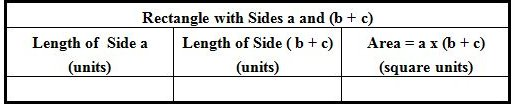1. What is the length of Side a?

2. What is the length of Side (b + c)?

3. What is the area of Rectangle 1 with Side a and Side (b + c)?

4. Complete the chart.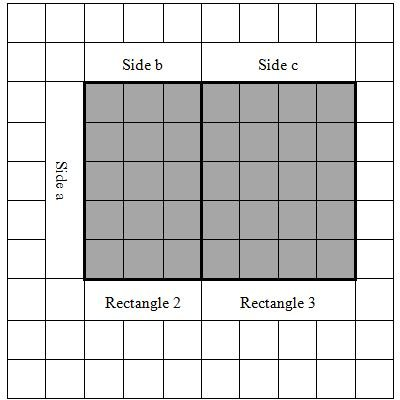Now look at Rectangle 2 and Rectangle 3 shown on the grid.

5. Rectangle 2 has Side a and Side b. Identify the lengths of the two sides of Rectangle 2.

6. Rectangle 3 has Side a and Side c. Identify the lengths of the two sides of Rectangle 3.

7. Find the areas of Rectangle 2 and Rectangle 3.

8. Complete the chart.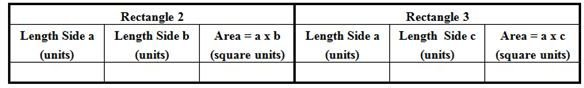9. What is the area of Rectangle 1?

10. What is the sum of the areas of Rectangle 2 and Rectangle 3?

11. Compare the area of Rectangle 1 with sides a and (b + c) and the sum of the areas of Rectangle 2 and Rectangle 3.

12. Write a statement about the comparison.

## Answers:

1. 5 units

2. 7 units

3. 35 square units

4. Complete the chart.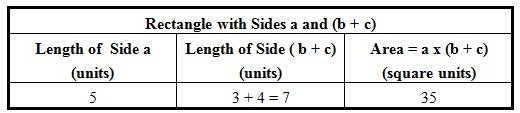5. 5 units and 3 units

6. 5 units and 4 units

7. 15 square units and 20 square units

8. Complete the chart.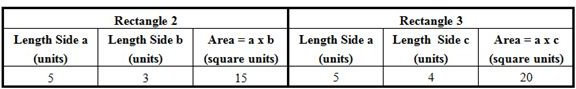9. 35 square units

10. 15 + 20 = 35 square units

11. The area of Rectangle 1 with sides 5 and (3 + 4) is 35. The sum of the areas of Rectangle 2, 15 square units, and Rectangle 3, 20 square units, is 35 square units.

12. The area of Rectangle 1 is equal to the sum of the areas of Rectangle 2 and Rectangle 3.

## Download Lesson with Individual or Group Work

To view the Individual or Group Work segment of this lesson, please download it here. Group work is at the end of the lesson.

## This post is part of the series: Grade 3 Math Lessons on Geometric Measurement

This series of lessons covers the concepts of geometric measurement for 3rd grade students. Through these lessons, students will understand concepts of area and relate area to multiplication and addition. Fulfills Common Core objectives.